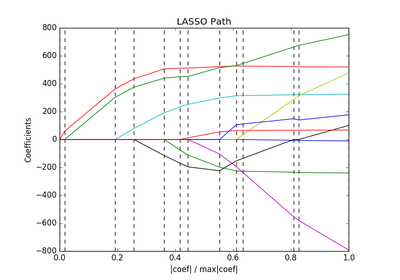# `sklearn.linear_model`.lars_path¶

`sklearn.linear_model.``lars_path`(X, y, Xy=None, Gram=None, max_iter=500, alpha_min=0, method='lar', copy_X=True, eps=2.2204460492503131e-16, copy_Gram=True, verbose=0, return_path=True, return_n_iter=False, positive=False)[source]

Compute Least Angle Regression or Lasso path using LARS algorithm 

The optimization objective for the case method=’lasso’ is:

```(1 / (2 * n_samples)) * ||y - Xw||^2_2 + alpha * ||w||_1
```

in the case of method=’lars’, the objective function is only known in the form of an implicit equation (see discussion in )

Read more in the User Guide.

Parameters: X : array, shape: (n_samples, n_features) Input data. y : array, shape: (n_samples) Input targets. positive : boolean (default=False) Restrict coefficients to be >= 0. When using this option together with method ‘lasso’ the model coefficients will not converge to the ordinary-least-squares solution for small values of alpha (neither will they when using method ‘lar’ ..). Only coefficients up to the smallest alpha value (```alphas_[alphas_ > 0.].min()``` when fit_path=True) reached by the stepwise Lars-Lasso algorithm are typically in congruence with the solution of the coordinate descent lasso_path function. max_iter : integer, optional (default=500) Maximum number of iterations to perform, set to infinity for no limit. Gram : None, ‘auto’, array, shape: (n_features, n_features), optional Precomputed Gram matrix (X’ * X), if `'auto'`, the Gram matrix is precomputed from the given X, if there are more samples than features. alpha_min : float, optional (default=0) Minimum correlation along the path. It corresponds to the regularization parameter alpha parameter in the Lasso. method : {‘lar’, ‘lasso’}, optional (default=’lar’) Specifies the returned model. Select `'lar'` for Least Angle Regression, `'lasso'` for the Lasso. eps : float, optional (default=``np.finfo(np.float).eps``) The machine-precision regularization in the computation of the Cholesky diagonal factors. Increase this for very ill-conditioned systems. copy_X : bool, optional (default=True) If `False`, `X` is overwritten. copy_Gram : bool, optional (default=True) If `False`, `Gram` is overwritten. verbose : int (default=0) Controls output verbosity. return_path : bool, optional (default=True) If `return_path==True` returns the entire path, else returns only the last point of the path. return_n_iter : bool, optional (default=False) Whether to return the number of iterations. alphas : array, shape: [n_alphas + 1] Maximum of covariances (in absolute value) at each iteration. `n_alphas` is either `max_iter`, `n_features` or the number of nodes in the path with `alpha >= alpha_min`, whichever is smaller. active : array, shape [n_alphas] Indices of active variables at the end of the path. coefs : array, shape (n_features, n_alphas + 1) Coefficients along the path n_iter : int Number of iterations run. Returned only if return_n_iter is set to True.

References

 [R182] “Least Angle Regression”, Effron et al. http://statweb.stanford.edu/~tibs/ftp/lars.pdf

## Examples using `sklearn.linear_model.lars_path`¶Lasso path using LARS